# Balbharati solutions for Chemistry 11th Standard Maharashtra State Board chapter 10 - States of Matter [Latest edition]

#### Chapters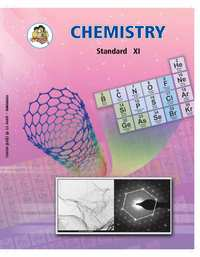## Chapter 10: States of Matter

Exercises
Exercises [Pages 157 - 159]

### Balbharati solutions for Chemistry 11th Standard Maharashtra State Board Chapter 10 States of Matter Exercises [Pages 157 - 159]

Exercises | Q 1. (A) | Page 157

Select and write the most appropriate alternatives from the given choices.

The unit of viscosity is

• dynes

• newton

• gram

• poise

Exercises | Q 1. (B) | Page 157

Select and write the most appropriate alternatives from the given choices.

Which of the following is true for 2 moles of an ideal gas?

• PV = nRT

• PV = RT

• PV = 2RT

• PV = T

Exercises | Q 1. (C) | Page 157

Select and write the most appropriate alternatives from the given choices.

Intermolecular forces in liquid are -

• greater than gases

• less than solids

• greater than gases and less than solids

• greater than solids

Exercises | Q 1. (D) | Page 157

Select and write the most appropriate alternatives from the given choices.

Interactive forces are __________ in an ideal gas.

• nil

• small

• large

• same as that of real gases

Exercises | Q 1. (E) | Page 157

Select and write the most appropriate alternatives from the given choices.

At constant temperature the pressure of 22.4 dm3 volume of an ideal gas was increased from 105 kPa to 210 kPa, New volume could be -

• 44.8 dm3

• 11.2 dm3

• 22.4 dm3

• 5.6 dm3

Exercises | Q 2. (A) | Page 157

Name the term used for the mixing of different gases by random molecular motion and frequent collision.

Exercises | Q 2. (B) | Page 157

The pressure that each individual gas would exert if it were alone in the container, what do we call it as?

Exercises | Q 2. (C) | Page 157

When a gas is heated the particles move more quickly. What is the change in the volume of a heated gas if the pressure is kept constant?

Exercises | Q 2. (D) | Page 157

A bubble of methane gas rises from the bottom of the North sea. What will happen to the size of the bubble as it rises to the surface?

Exercises | Q 2. (E)(a) | Page 157

Convert the following temperature from degree Celcius to kelvin.

−15° C

Exercises | Q 2. (E)(b) | Page 157

Convert the following temperature from degree Celcius to kelvin.

25° C

Exercises | Q 2. (E)(c) | Page 157

Convert the following temperature from degree Celcius to kelvin.

−197° C

Exercises | Q 2. (E)(d) | Page 157

Convert the following temperature from degree Celcius to kelvin.

273° C

Exercises | Q 2. (F)(a) | Page 157

Convert the following pressure value into Pascals.

10 atmosphere

Exercises | Q 2. (F)(b) | Page 157

Convert the following pressure value into Pascals.

1 kPa

Exercises | Q 2. (F)(c) | Page 157

Convert the following pressure value into Pascals.

107000 Nm−2

Exercises | Q 2. (F)(d) | Page 157

Convert the following pressure value into Pascals.

1 atmosphere

Exercises | Q 2. (G)(a) | Page 157

Convert exactly 1.5 atm to pascals

Exercises | Q 2. (G)(b) | Page 157

Convert 89 kPa to newton per square metre (Nm−2)

Exercises | Q 2. (G)(c) | Page 157

Convert 101.325 kPa to bar.

Exercises | Q 2. (G)(d) | Page 157

Convert −100° C to kelvin

Exercises | Q 2. (G)(e) | Page 157

Convert 0.124 torr to the standard atmosphere

Exercises | Q 2. (H) | Page 157

If the density of a gas is measured at constant temperature and pressure then which of the following statement is correct?

• Density is directly proportional to molar mass of the gas.

• Greater the density greater is the molar mass of the gas.

• If density, temperature and pressure is given ideal gas equation can be used to find molar mass.

• All the above statements are correct.

Exercises | Q 2. (I)(a) | Page 157

Observe the following conversion.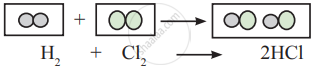Is the above reaction is in accordance with the principle of stoichiometry?

Exercises | Q 2. (I)(b) | Page 157

Observe the following conversion.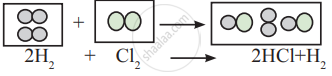Is the above reaction is in accordance with the principle of stoichiometry?

Exercises | Q 2. (J) | Page 157

Hot air balloons float in the air because of the low density of the air inside the balloon. Explain this with the help of an appropriate gas law.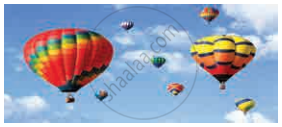Exercises | Q 3. (A)(a) | Page 158

Identify the gas laws from the following diagram.

 Diagram Gas laws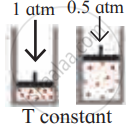______________
Exercises | Q 3. (A)(b) | Page 158

Identify the gas laws from the following diagram.

 Diagram Gas laws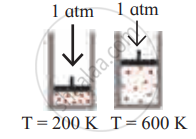______________
Exercises | Q 3. (A)(c) | Page 158

Identify the gas laws from the following diagram.

 Diagram Gas laws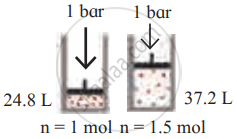______________
Exercises | Q 3. (B)(a) | Page 158

Consider a sample of a gas in a cylinder with a movable piston.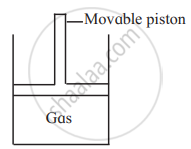Show diagrammatically the changes in the position of the piston, if pressure is increased from 1.0 bar to 2.0 bar at a constant temperature.

Exercises | Q 3. (B)(b) | Page 158

Consider a sample of a gas in a cylinder with a movable piston.Show diagrammatically the changes in the position of the piston, if the temperature is decreased from 300 K to 150 K at constant pressure.

Exercises | Q 3. (B)(c) | Page 158

Consider a sample of a gas in a cylinder with a movable piston.Show diagrammatically the changes in the position of the piston, if the temperature is decreased from 400 K to 300 K, and pressure is decreased from 4 bar to 3 bar.

Exercises | Q 3. (C) | Page 158

List the characteristic physical properties of the gases.

Exercises | Q 3. (D)(a) | Page 158

Define the term polarizability

Exercises | Q 3. (D)(b) | Page 158

Define the term Hydrogen bond

Exercises | Q 3. (D)(c) | Page 158

Define the term Aqueous tension

Exercises | Q 3. (D)(d) | Page 158

Define the term Dipole moment

Exercises | Q 3. (E) | Page 158

Would it be easier to drink water with a straw on the top of Mount Everest or at the base? Explain.

Exercises | Q 3. (F)(a) | Page 158

Identify type of the intermolecular forces in the following compound.

CH3-OH

Exercises | Q 3. (F)(b) | Page 158

Identify type of the intermolecular forces in the following compound.

CH2=CH2

Exercises | Q 3. (F)(c) | Page 158

Identify type of the intermolecular forces in the following compound.

CHCl3

Exercises | Q 3. (F)(d) | Page 158

Identify type of the intermolecular forces in the following compound.

CH2Cl2

Exercises | Q 3. (G) | Page 158

Name the types of intermolecular forces present in Ar.

Exercises | Q 3. (G) | Page 158

Name the types of intermolecular forces present in Cl2.

Exercises | Q 3. (G) | Page 158

Name the types of intermolecular forces present in CCl4.

Exercises | Q 3. (G) | Page 158

Name the types of intermolecular forces present in HNO3.

Exercises | Q 3. (H) | Page 158

Match the pairs of the following:

 Column ‘A’ Column ‘B’ a. Boyle’s law i. at constant pressure and volume b. Charles’ law ii. at constant temperature iii. at constant pressure
Exercises | Q 3. (I)(a) | Page 158

Write the statement for Boyle’s law

Exercises | Q 3. (I)(b) | Page 158

Write the statement for Charles’ law

Exercises | Q 3. (J) | Page 158

Differentiate between Real gas and Ideal gas.

Exercises | Q 4. (A) | Page 158

State and write the mathematical expression for Dalton’s law of partial pressure and explain it with a suitable example.

Exercises | Q 4. (B) | Page 158

Derive an Ideal gas equation. Mention the terms involved in it.

Exercises | Q 4. (B) | Page 158

Write how ideal gas equation is utilised to obtain combined gas law.

Exercises | Q 4. (C)(a) | Page 158

With the help of the graph answer the following -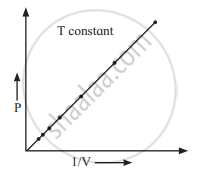At constant temperature, the Graph shows the relationship between pressure and volume. Represent the relation mathematically.

Exercises | Q 4. (C)(b) | Page 158

With the help of the graph answer the following -At constant temperature, Identify the law.

Exercises | Q 4. (C)(c) | Page 158

With the help of the graph answer the following -At constant temperature, Write the statement of law.

Exercises | Q 4. (D) | Page 158

Write Postulates of the kinetic theory of gases.

Exercises | Q 4. (E)(a) | Page 158

Write a short note on Vapour.

Exercises | Q 4. (E)(b) | Page 158

Write a short note on Surface tension.

Exercises | Q 4. (E)(c) | Page 158

Write a short note on Viscosity.

Exercises | Q 5. (A) | Page 159

Solve the following.

A balloon is inflated with helium gas at room temperature of 25°C and at 1 bar pressure when its initial volume is 2.27L and allowed to rise in the air. As it rises in the air external pressure decreases and the volume of the gas increases till finally, it bursts when external pressure is 0.3bar. What is the limit at which the volume of the balloon can stay inflated?

Exercises | Q 5. (B) | Page 159

Solve the following.

A syringe has a volume of 10.0 cm3 at pressure 1 atm. If you plug the end so that no gas can escape and push the plunger down, what must be the final volume to change the pressure to 3.5 atm?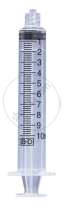Exercises | Q 5. (C)(a) | Page 159

Solve the following.

The volume of a given mass of a gas at 0°C is 2 dm3. Calculate the new volume of the gas at constant pressure when the temperature is increased by 10°C.

Exercises | Q 5. (C)(b) | Page 159

Solve the following.

The volume of a given mass of a gas at 0°C is 2 dm3. Calculate the new volume of the gas at constant pressure when the temperature is decreased by 10°C.

Exercises | Q 5. (D) | Page 159

Solve the following.

A hot air balloon has a volume of 2800 m3 at 99°C. What is the volume if the air cools to 80°C?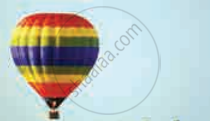Exercises | Q 5. (E) | Page 159

Solve the following.

At 0°C, a gas occupies 22.4 liters. How much hot must be the gas in celsius and in kelvin to reach a volume of 25.0 liters?

Exercises | Q 5. (F) | Page 159

Solve the following.

A 20 L container holds 0.650 mol of He gas at 37°C at a pressure of 628.3 bar. What will be new pressure inside the container if the volume is reduced to 12 L. The temperature is increased to 177°C and 1.25 mol of additional He gas was added to it?

Exercises | Q 5. (G) | Page 159

Solve the following.

Nitrogen gas is filled in a container of volume 2.32 L at 32°C and 4.7 atm pressure. Calculate the number of moles of the gas.

Exercises | Q 5. (H) | Page 159

Solve the following.

At 25°C and 760 mm of Hg pressure, a gas occupies 600 mL volume. What will be its pressure at the height where the temperature is 10°C and the volume of the gas 640 mL?

Exercises | Q 5. (I) | Page 159

Solve the following.

A neon-dioxygen mixture contains 70.6 g dioxygen and 167.5g neon. If the pressure of the mixture of the gases in the cylinder is 25 bar. What is the partial pressure of dioxygen and neon in the mixture?

Exercises | Q 5. (J) | Page 159

Solve the following.

Calculate the pressure in atm of 1.0 mole of helium in a 2.0 dm3 container at 20.0°C.

Exercises | Q 5. (K) | Page 159

Solve the following.

Calculate the volume of 1 mole of a gas at exactly 20°C at a pressure of 101.35 kPa.

Exercises | Q 5. (L) | Page 159

Solve the following.

Calculate the number of molecules of methane in 0.50 m3 of the gas at a pressure of 2.0 × 102 kPa and a temperature of exactly 300 K.

## Chapter 10: States of Matter

Exercises## Balbharati solutions for Chemistry 11th Standard Maharashtra State Board chapter 10 - States of Matter

Balbharati solutions for Chemistry 11th Standard Maharashtra State Board chapter 10 (States of Matter) include all questions with solution and detail explanation. This will clear students doubts about any question and improve application skills while preparing for board exams. The detailed, step-by-step solutions will help you understand the concepts better and clear your confusions, if any. Shaalaa.com has the Maharashtra State Board Chemistry 11th Standard Maharashtra State Board solutions in a manner that help students grasp basic concepts better and faster.

Further, we at Shaalaa.com provide such solutions so that students can prepare for written exams. Balbharati textbook solutions can be a core help for self-study and acts as a perfect self-help guidance for students.

Concepts covered in Chemistry 11th Standard Maharashtra State Board chapter 10 States of Matter are Introduction of States of Matter: Gaseous and Liquid States, Intermolecular Forces, Characteristic Properties of Gases, The Gas Laws, Ideal Gas Equation, Kinetic Molecular Theory of Gases, Deviation from Ideal Behaviour, Liquefaction of Gases and Critical Constant, Liquid State.

Using Balbharati 11th solutions States of Matter exercise by students are an easy way to prepare for the exams, as they involve solutions arranged chapter-wise also page wise. The questions involved in Balbharati Solutions are important questions that can be asked in the final exam. Maximum students of Maharashtra State Board 11th prefer Balbharati Textbook Solutions to score more in exam.

Get the free view of chapter 10 States of Matter 11th extra questions for Chemistry 11th Standard Maharashtra State Board and can use Shaalaa.com to keep it handy for your exam preparation